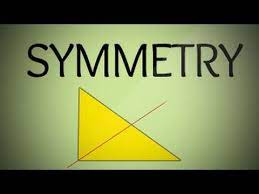# Symmetry: Two or More Parts of a Figure are Identical after Folding or Flipping

In this quiz, you will find questions based on symmetric objects, different types of symmetry, etc.Start Quiz

How many lines of symmetry are there in a rhombus?

1 2 3 4

How many lines of symmetry are there in a scalene triangle?

1 0 2 4

How many lines of symmetry are there in an isosceles triangle?

4 3 1 2

How many lines of symmetry are there in a rectangle?

1 2 3 4

State the number of lines of symmetry for a parallelogram.

0 1 2 None of these

Which of the following triangles has no line of symmetry?

An equilateral triangle An isosceles triangle A scalene triangle All of the above

Which of these letters has only rotational symmetry?

S E B P

Which of the following figure has no line of symmetry

Parallelogram Isosceles triangle Circle Semicircle

Letter ‘H’ of the English alphabet have reflectional symmetry (i.e., symmetry related to mirror reflection) about.

A vertical mirror

Both horizontal and vertical

A horizontal mirror

Neither horizontal nor vertical

A line of symmetry is also called a

Mirror line

Vertical line

Perpendicular line

Horizontal line

In a circle every ________ is a line of symmetry

Diameter

Both

Chord

None of all

The number of lines of symmetry of a circle is

Unlimited

8

16

4

Which of the following has only one line of symmetry?

A rectangle

A square

A semi circle

An equilateral triangle

How many lines of symmetry are there in a regular pentagon?

1

2

3

5

How many lines of symmetry are there in an irregular quadrilateral?

0

2

4

None of these

Quiz/Test Summary
Title: Symmetry: Two or More Parts of a Figure are Identical after Folding or Flipping
Questions: 15
Contributed by: